﻿ 玄武岩纤维活性粉末混凝土力学性能试验研究

玄武岩纤维活性粉末混凝土力学性能试验研究Experimental Study on Mechanical Properties of Basalt Fiber Reinforced Reactive Powder Concrete

Abstract: Basalt fiber reinforced reactive powder concrete (RPC) has the advantages of high strength, good durability, and high temperature resistance, which is suitable for building structural materials under complex conditions. In order to study the static mechanical properties of basalt fiber reinforced RPC, 5 kinds of fiber volume content of 0.1%, 0.15%, 0.20%, 0.25%, 0.30% and two different fiber lengths of 6 mm and 12 mm of basalt fibers were considered. The influence of basalt fiber on the compressive strength and flexural strength of RPC was studied, and the test results of other basalt fiber reinforced concrete were compared and analyzed. The results show that basalt fiber has little effect on improving the compressive strength, and even shows a negative growth, but the effect on flexural strength is more obvious. As the content of basalt fiber increases, the flexural strength first increases and then decreases. The best volume content of basalt fiber is 0.15%~0.20%. The fiber with a length of 6 mm is better than the fiber with a length of 12 mm in improving the compressive strength and flexural strength. Through comparative analysis, it is found that the influence of basalt fiber on compressive strength is not obvious, but is better on improving the flexural strength. The research of this paper can provide a certain theoretical basis for the application of basalt fiber reinforced RPC.

1. 引言

2. 试验概况

2.1. 原材料与配合比Table 1. Mix ratio for experiment

2.2. 试件制备

2.3. 试验方法Figure 1. 200 t electronic universal testing machine (compression test)Figure 2. 100 t electronic universal testing machine (flexural test)

3. 试验结果与分析

3.1. 试验现象

3.1.1. 抗压试验现象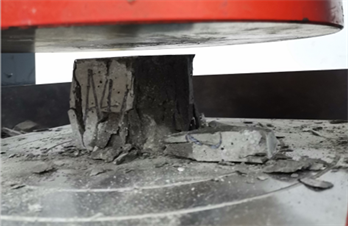Figure 3. The shape of the compressive specimen after failure

3.1.2. 抗折试验现象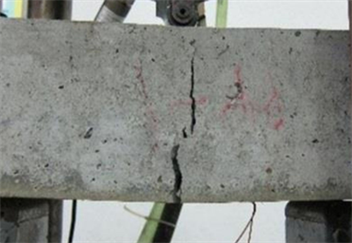Figure 4. Form after failure of flexural specimen

3.2. 抗压强度

${f}_{cu}^{6}=112.84-159.18{V}_{f}^{6}\text{ }\text{ }\text{ }\text{ }\text{ }\text{ }\text{ }\text{ }\text{ }\text{ }\text{ }\text{ }\text{ }\text{ }\text{ }\text{ }\text{ }\text{ }\text{ }\text{ }\text{ }\text{ }0.1%\le {V}_{f}^{6}\text{ }\le 0.3%\text{ }\text{ }\text{ }\text{ }\text{ }\text{ }\text{ }\text{ }\text{ }\text{ }\text{ }\text{ }\text{ }\text{ }\text{ }\text{ }\text{ }\text{ }{R}^{2}=0.9656$ (1)

${f}_{cu}^{12}=88.41-78.66{V}_{f}^{12}\text{ }\text{ }\text{ }\text{ }\text{ }\text{ }\text{ }\text{ }\text{ }\text{ }\text{ }\text{ }\text{ }\text{ }\text{ }\text{ }\text{ }\text{ }\text{ }\text{ }\text{ }\text{ }0.1%\le {V}_{f}^{12}\text{ }\le 0.3%\text{ }\text{ }\text{ }\text{ }\text{ }\text{ }\text{ }\text{ }\text{ }\text{ }\text{ }\text{ }\text{ }\text{ }\text{ }\text{ }\text{ }\text{ }{R}^{2}=0.9467$ (2)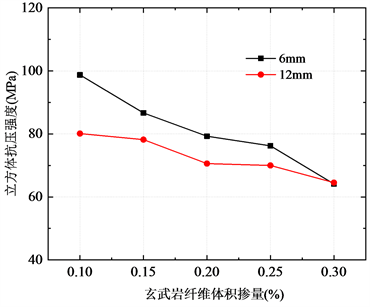(a) 实测抗压强度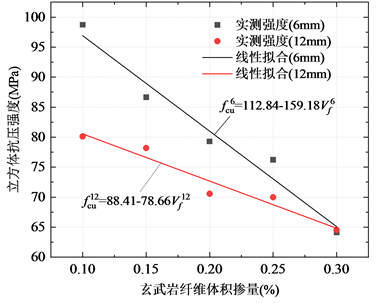(b) 线性拟合曲线

Figure 5. Cube compressive strength changes with fiber content

3.3. 抗折强度

${f}_{f}^{6}=\left\{\begin{array}{c}11.4+20{V}_{f}^{6}\text{ }\text{ }\text{ }\text{ }\text{ }\text{ }\text{ }\text{ }\text{ }\text{ }\text{ }\text{ }\text{ }\text{ }\text{ }\text{ }\text{ }\text{ }\text{ }\text{ }\text{ }\text{ }\text{ }\text{ }\text{ }\text{ }\text{ }\text{ }\text{ }\text{ }\text{ }\text{ }\text{ }\text{ }\text{ }\text{ }\text{ }0.1%\le {V}_{f}^{6}\text{ }\le 0.2%\text{ }\text{ }\text{ }\text{ }\text{ }\text{ }\text{ }\text{ }\text{ }\text{ }\text{ }\text{ }\text{ }\text{ }\text{ }\text{ }\text{ }\text{ }{R}^{2}=0.971\\ 20.31-24.5{V}_{f}^{6}\text{ }\text{ }\text{ }\text{ }\text{ }\text{ }\text{ }\text{ }\text{ }\text{ }\text{ }\text{ }\text{ }\text{ }\text{ }\text{ }\text{ }\text{ }\text{ }\text{ }\text{ }\text{ }0.2%\le {V}_{f}^{6}\text{ }\le 0.3%\text{ }\text{ }\text{ }\text{ }\text{ }\text{ }\text{ }\text{ }\text{ }\text{ }\text{ }\text{ }\text{ }\text{ }\text{ }\text{ }\text{ }\text{ }{R}^{2}=0.983\end{array}$ (3)

${f}_{f}^{12}=\left\{\begin{array}{c}8.82+30{V}_{f}^{12}\text{ }\text{ }\text{ }\text{ }\text{ }\text{ }\text{ }\text{ }\text{ }\text{ }\text{ }\text{ }\text{ }\text{ }\text{ }\text{ }\text{ }\text{ }\text{ }\text{ }\text{ }\text{ }\text{ }\text{ }\text{ }\text{ }\text{ }\text{ }\text{ }\text{ }\text{ }\text{ }\text{ }\text{ }\text{ }\text{ }\text{ }0.1%\le {V}_{f}^{12}\text{ }\le 0.2%\text{ }\text{ }\text{ }\text{ }\text{ }\text{ }\text{ }\text{ }\text{ }\text{ }\text{ }\text{ }\text{ }\text{ }\text{ }\text{ }\text{ }\text{ }{R}^{2}=0.957\\ 18.88-20.5{V}_{f}^{12}\text{ }\text{ }\text{ }\text{ }\text{ }\text{ }\text{ }\text{ }\text{ }\text{ }\text{ }\text{ }\text{ }\text{ }\text{ }\text{ }\text{ }\text{ }\text{ }\text{ }\text{ }\text{ }0.2%\le {V}_{f}^{12}\text{ }\le 0.3%\text{ }\text{ }\text{ }\text{ }\text{ }\text{ }\text{ }\text{ }\text{ }\text{ }\text{ }\text{ }\text{ }\text{ }\text{ }\text{ }\text{ }\text{ }{R}^{2}=0.874\end{array}$ (4)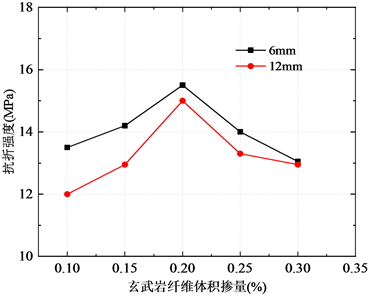(a) 实测抗折强度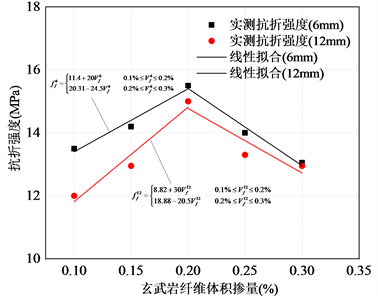(b) 线性拟合曲线

Figure 6. Flexural strength changes with fiber content

4. 试验结果对比分析Figure 7. Comparison of compressive strength test results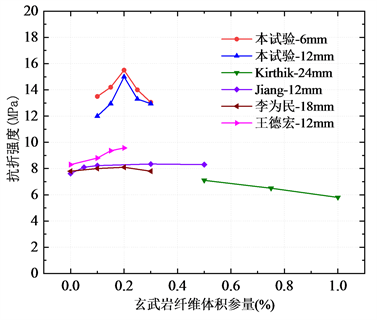Figure 8. Comparison of flexural strength test results

5. 结论

 郑文忠, 吕雪源. 活性粉末混凝土研究进展[J]. 建筑结构学报, 2015, 36(10): 44-58.

 Li, H.Y. and Liu, G. (2016) Tensile Properties of Hybrid Fiber-Reinforced Reactive Powder Concrete after Exposure to Elevated Temperatures. International Journal of Concrete Structures and Materials, 10, 29-37.
https://doi.org/10.1007/s40069-016-0125-z

 侯敏, 陶燕, 陶忠, 柴栋. 关于玄武岩纤维混凝土的增强机理研究[J]. 混凝土, 2020(2): 67-71+75.

 Kirthika, S.K. and Singh, S.K. (2018) Experimental Investigations on Basalt Fibre-Reinforced Concrete. Journal of the Institution of Engineers (India): Series A, 99, 661-670.
https://doi.org/10.1007/s40030-018-0325-4

 Kabay, N. (2014) Abrasion Resistance and Fracture Energy of Concretes with Basalt Fiber. Construction and Building Materials, 50, 95-101.
https://doi.org/10.1016/j.conbuildmat.2013.09.040

 李为民, 许金余, 沈刘军, 等. 玄武岩纤维混凝土的动态力学性能[J]. 复合材料学报, 2008, 25(2): 135-142.

 Jiang, C.H., Fan, K., Wu, F. and Chen, D. (2014) Experimental Study on the Mechanical Properties and Microstructure of Chopped Basalt Fibre Reinforced Concrete. Materials and Design, 58, 187-193.
https://doi.org/10.1016/j.matdes.2014.01.056

 Wang, D.H., Ju, Y.Z., Shen, H. and Xu, L.B. (2019) Mechanical Properties of High Performance Concrete Reinforced with Basalt Fiber and Polypropylene Fiber. Construction and Building Materials, 197, 464-473.
https://doi.org/10.1016/j.conbuildmat.2018.11.181

Top